# Problem 46963. Roots, Bloody Roots: part 2/2

Using curves or lines, we can easily spot roots from n-degree polynomials in 2D: wherever they cross the real line, we know there is a root. However, as we are well aware, roots can also be complex numbers. In this case, plotting curves no longer seem adequate since they won't be found at the real line. That's when coloring comes into place; by painting the 2D plane, we can visualize where complex or real roots are and get a sense of what's happening in a function.
This problem is the second part of a previous one; if you haven't solved it, Problem 46833, please do it before proceeding. Finally, we will color the complex plane following the roots of any given polynomial using the color space HSV. The same rules for HSV-space apply, but instead of using the cube root for V, we will allow the user to choose its gamma correction parameters used to the Min-max normalization of the distance,, in which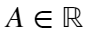and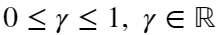. Once the three values are computed, convert them to RGB space using the function hsv2rgb and round them to 4 decimal places. Moreover, instead of plotting a matrix, m x n x 3, centered at the origin, we will center it at the bounding-box enveloping the roots of a given polynomial P; horizontal axis imaginary and vertical axis real.
Any line has only one root, which should always be at the center of the image (generating a figure similar to those obtained at the previous problem). As another example of expected output, this is the result for the 10th roots of unit given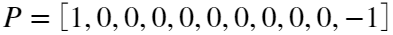,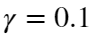,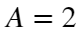,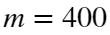and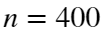.Polynomials may have any number of roots between 1 and the maximum that MATLAB can compute (or Cody), which means one or two dimensions may have thickness 0. Your algorithm must take this case into account by calculating the minimum bounding-box and doubling the distance from the box's center to its edges. If one size has thickness zero, use the same thickness as the non-zero one, and if both are zero, use a square of side 2 (a distance of two times one). The center of the box can be found using, in which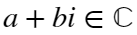. Since we render a matrix of size mxn, your algorithm should convert each pixel position to the complex roots' bounding box interval. This operation is linear, and you may use the function interp1. All values obtained by interpolation must also be rounded to 4 decimal places.
Good luck!
Note-1: This problem is inspired by a 3Blue1Brown's video: https://www.youtube.com/watch?v=b7FxPsqfkOY, and I recommend that you watch his video if you haven't already. However, it is not required that you do to solve this problem.
Note-2: This problem was last updated 22/08/2020. Thanks to Svyatoslav's feedback. Please, let me know if anyone run into issues.

### Solution Stats

11.11% Correct | 88.89% Incorrect
Last Solution submitted on Jul 02, 2021

### Community Treasure Hunt

Find the treasures in MATLAB Central and discover how the community can help you!

Start Hunting!